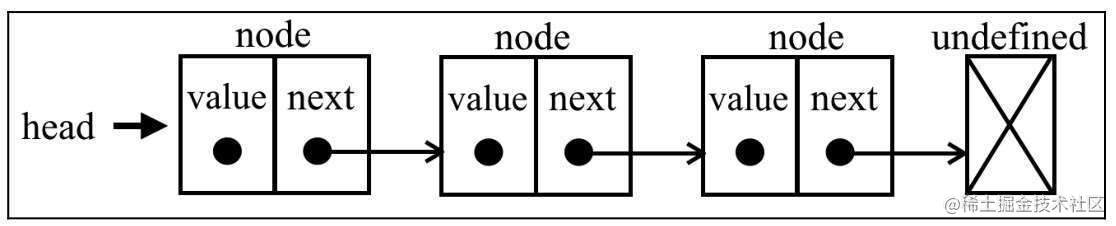# 认识链表

## 什么是链表## 实现一个链表

`class LinkedList {  constructor() {    this.count = 0;    this.head = undefined;  }}`

• `count`：表示链表的长度，也就是元素的个数
• `head`：存储第一个元素（表头）的引用

`class Node {  constructor(value) {    this.value = value;    this.next = undefined;  }}`

• `push`：向链表尾部添加一个元素
• `removeAt`：从链表某处移除一个元素

### push 实现

• 链表为空，则添加第一个元素
• 链表不为空，在所有元素之后添加元素
`push(item) {  let node = new Node(item)  let current;  if(!this.head) {    this.head = node  } else {    current = this.head    while(current.next) {      current = current.next    }    current.next = node;  }  this.count++;}`

### removeAt 实现

`removeAt(index) {  if(index >= 0 && index < this.count) {    // 具体逻辑  }  return undefined;}`

`if (index === 0) {  let current = this.head;  this.head = current.next;}`

1. 找到 index 对应的元素 B
2. 找到 B 的上一个元素 A
3. `A.next` 指向 `B.next`

`if (index > 0) {  let current = this.head;  let previous;  for (let i = 0; i < index; i++) {    previous = current;    current = current.next;  }}`

`removeAt(index) {  if(index >= 0 && index < this.count) {    let current = this.head;    // 第一项    if(index === 0) {      this.head = current.next;    } else {      let previous;      for(let i = 0; i < index; i++) {        previous = current;        current = current.next;      }      // 跳过 current，实现删除的目的      previous.next = current.next    }    this.count--;    return current.value  }  return undefined;}`